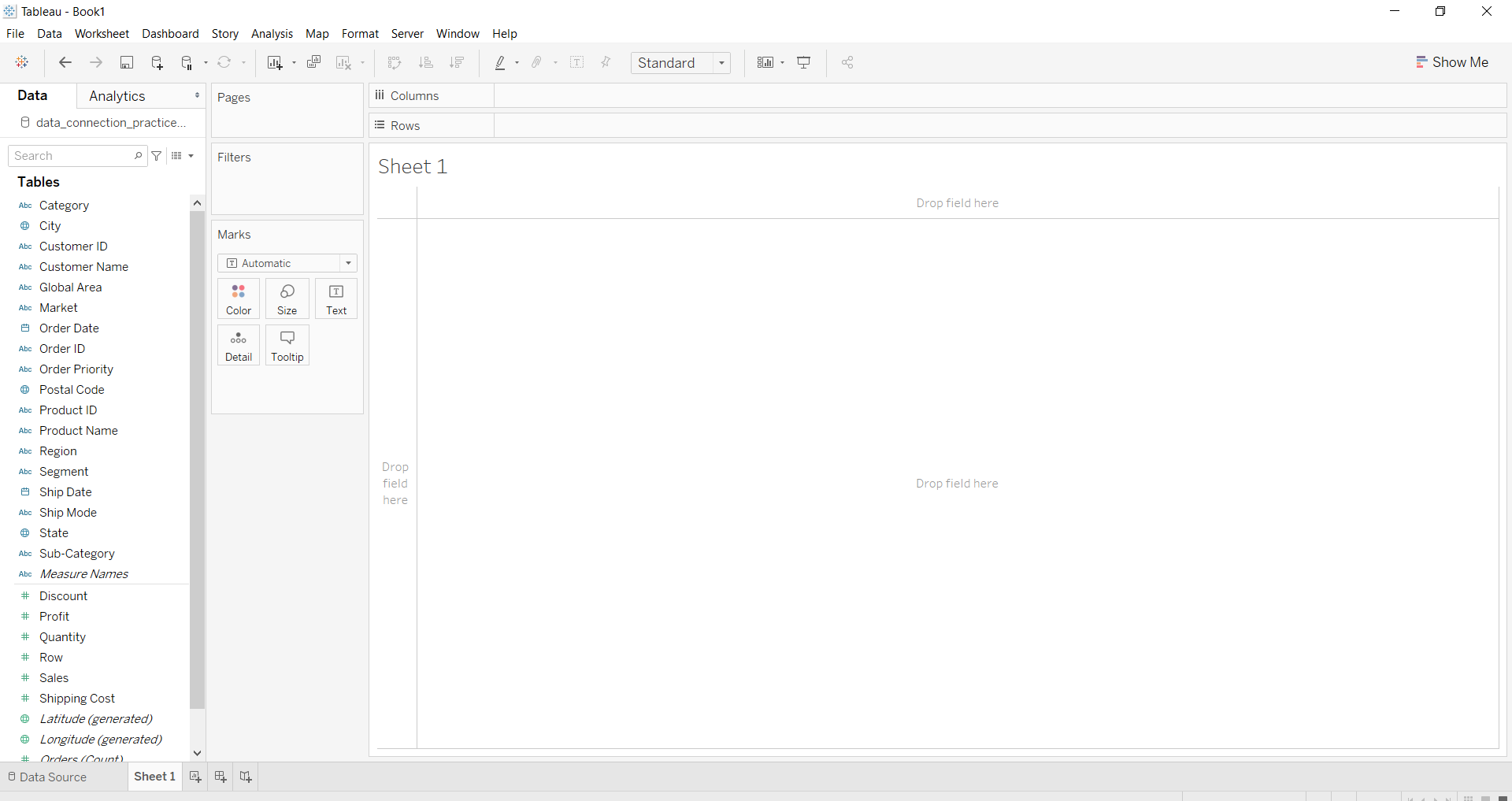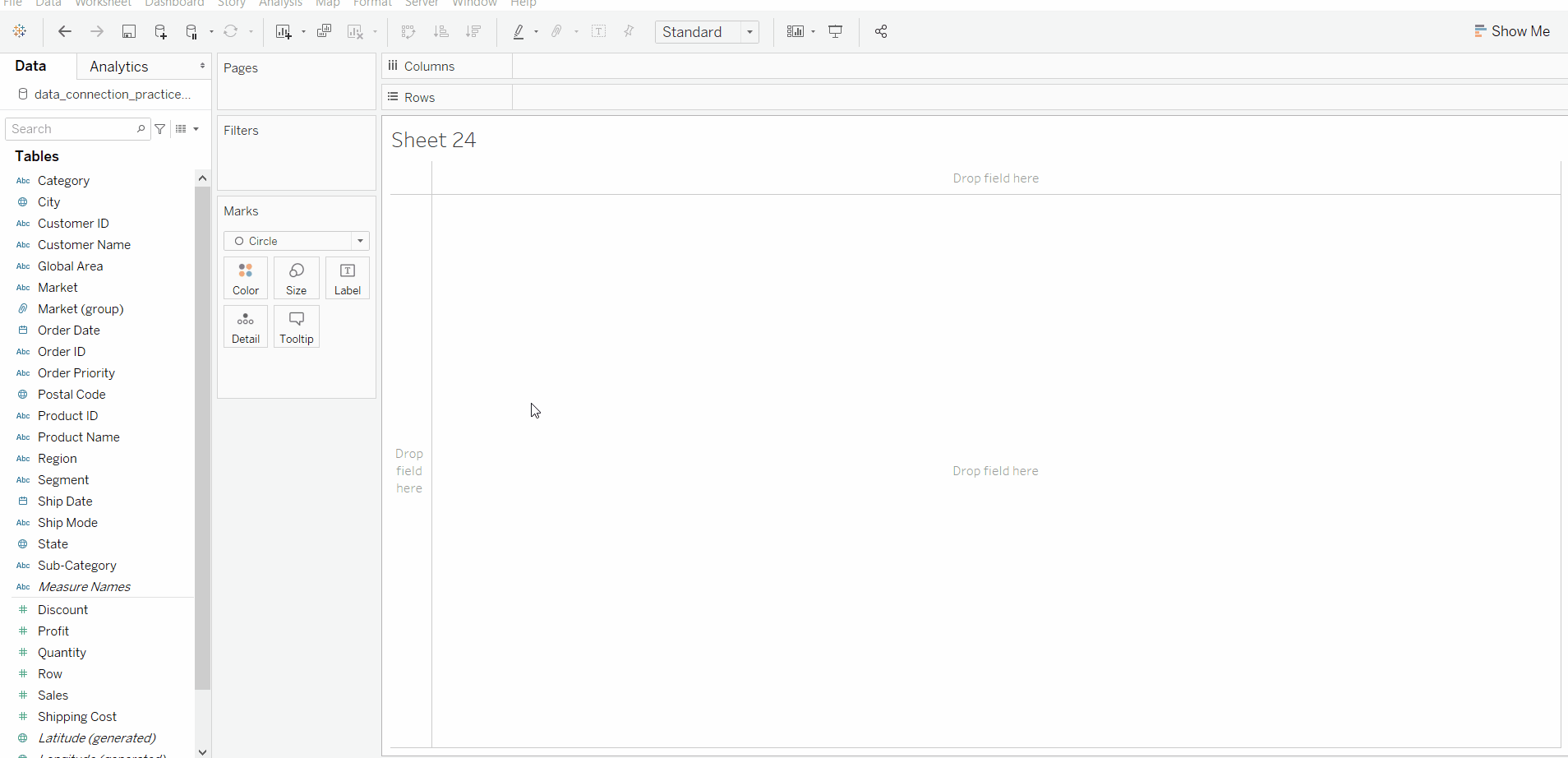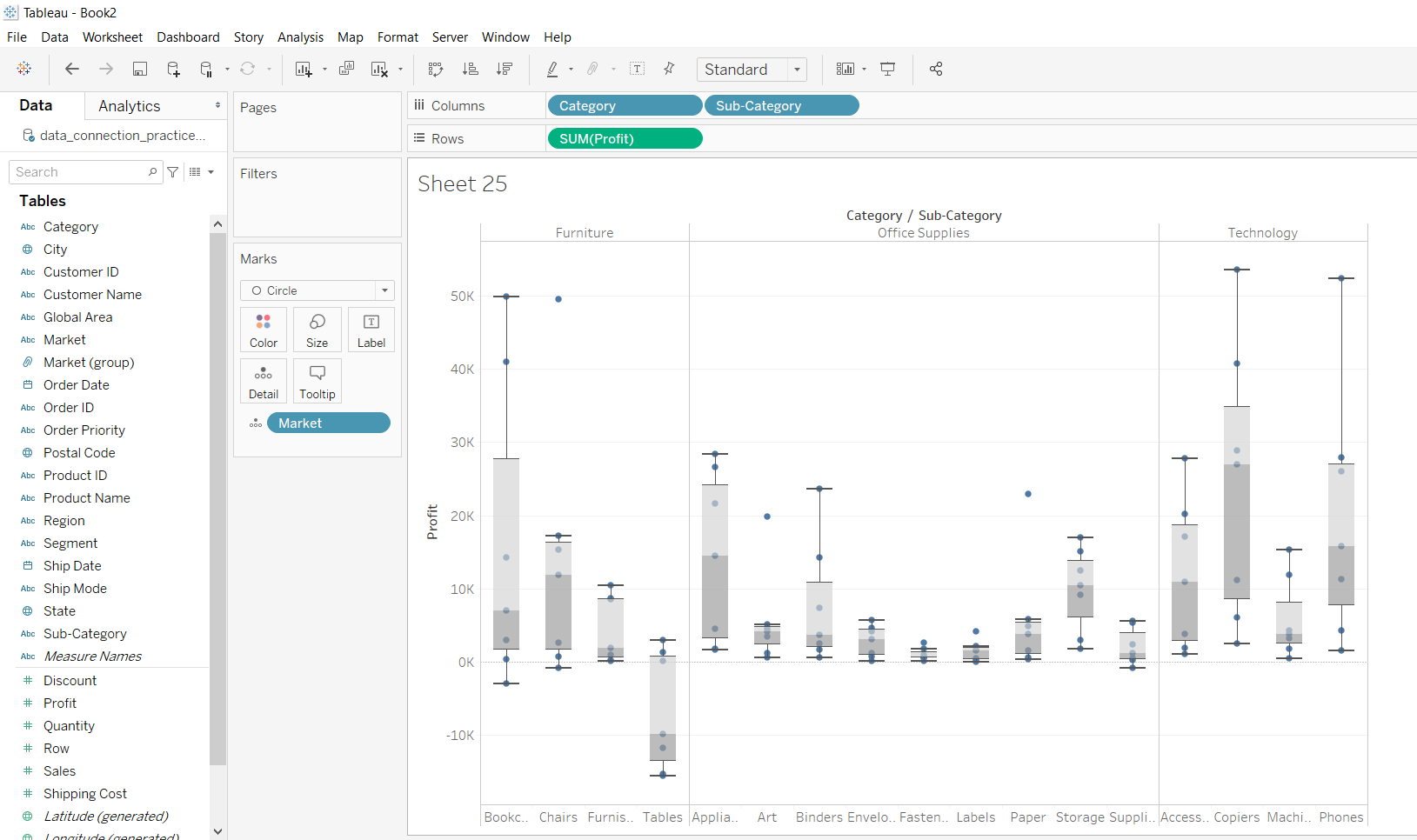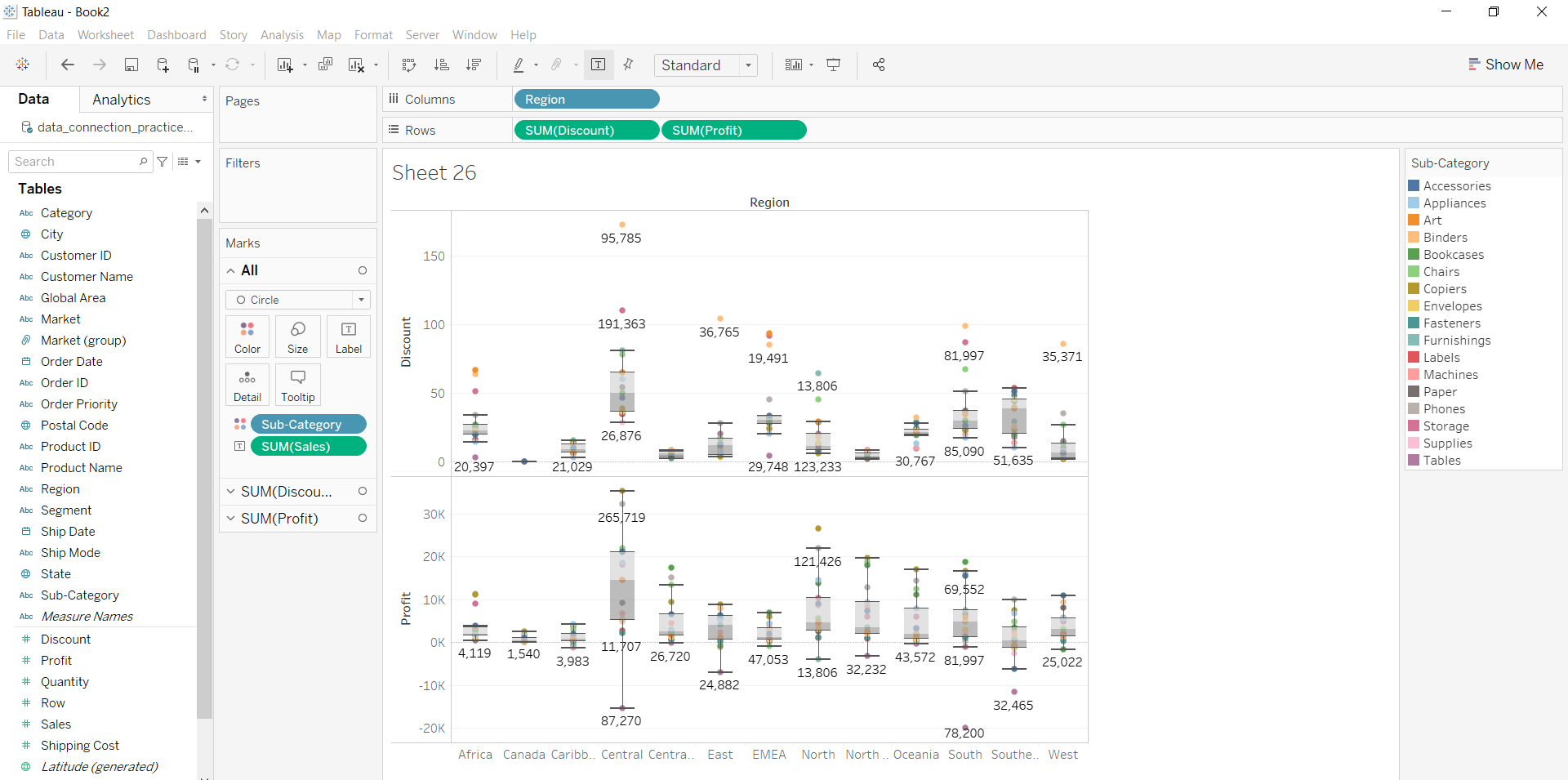Related Articles
Box Plot in Tableau
• Last Updated : 01 Oct, 2020

In this article, we will learn how to draw box plot in tableau worksheet to do further operations. For this first look into two terms :

• Tableau: Tableau is a very powerful data visualization tool that can be used by data analysts, scientists, statisticians, etc. to visualize the data and get a clear opinion based on the data analysis. Tableau is very famous as it can take in data and produce the required data visualization output in a very short time.
• Box Plot: It is the visual representation of the depicting groups of numerical data through their quartiles. Boxplot is also used for detecting the outlier in the data set. It captures the summary of the data efficiently with a simple box and whiskers and allows us to compare easily across groups.

Dataset used in the given examples is Dataset.

For this we have to follow some steps :

Open the Tableau tool and connect a dataset into it. Drag and drop the one sheet of the connected dataset. Click on sheet1 to open the tableau worksheet. On clicking Sheet1 you will get whole dataset attributes on the left side and a worksheet for work.To draw a Box Plot you have to select a minimum of three attributes (one in row, one in column and one for view) by drag and drop then select the chart option as box plot.

Example 1 :Example 2: This example is also drawn similar to above example 1 with some extra marks and which is explained in steps given below :

• Select the Category with sub-category and profit fields as column and row respectively.
• Select the chart type box plot.
• Apply marks by color of market.Example 3: This example is also drawn similar to above example 1 with some extra marks and which is explained in steps given below:

• Select the Region and Discount with profit fields as column and row respectively.
• Select the chart type box plot.
• Apply marks by color of sub-category.
• Apply marks by text of sales.My Personal Notes arrow_drop_up